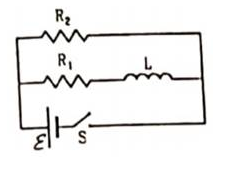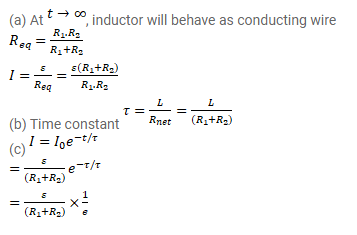# Consider the circuit shown in figure.

Question:

Consider the circuit shown in figure.

(a) Find the current through the battery a long time after the switch $\mathrm{S}$ is closed.

(b) Suppose the switch is again opened at $\mathrm{t}=0$. What is the time constant of the discharging circuit?

(c) Find the current through the inductor after one time constant.Solution: